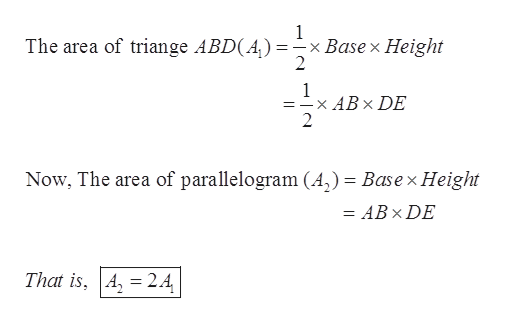# If both a parallelogram and triangle have the same base and altitude, then the area of the parallelogram is twice the area of the triangle. True or false

Question
1 views

If both a parallelogram and triangle have the same base and altitude, then the area of the parallelogram is twice the area of the triangle. True or false

check_circle

Step 1

Given: If both a parallelogram and triangle have the same base and altitude, then the area of the parallelogram is twice the area of the triangle.

Step 2

As below, Parallelogram ABCD and ∆ABD are on the same base AB and the same altitude DE.

Step 3

Now,

...help_outlineImage Transcriptionclose1 x Base x Height 2 The area of triange ABD(A) : 1 =-x ABx DE 2 Now, The area of parallelogram (A,) = Basex Height = ABx DE That is, A 24 fullscreen

### Want to see the full answer?

See Solution

#### Want to see this answer and more?

Solutions are written by subject experts who are available 24/7. Questions are typically answered within 1 hour.*

See Solution
*Response times may vary by subject and question.
Tagged in

### Triangles